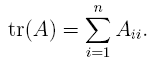## Tuesday, September 15, 2009

### Trace Tricks

The trace of a square matrix is the sum of the elements on the main diagonal. That is, for an n by n square matrix A, the trace of A isThis might not seem too exciting at first. However, the trace operator has a neat quasi-commutative property: for matrices U and V, so long as the internal dimensions work out, it is true thatThe proof isn't too hard so I'll skip it. If we had a third matrix W (again assuming the internal dimensions work out), since matrix multiplication is associative, it is also true thatIt's not truly commutative, since you can only do cyclic shifts of the arguments. So, e.g., tr(UVW) is not equal to tr(WVU) in general.

What can you do with this? For one thing, note that the trace of a scalar a is itself: tr(a) = a. So if you have a matrix multiplication that results in a scalar, you can use trace to rearrange the arguments.

For instance, let U be a 1 by n row vector, and let V be an n by n matrix. If U' is the transpose of U, then UVU' is a scalar. This kind of expression comes up pretty often in jointly Gaussian distributions.

Now say U is a zero-mean vector with covariance matrix E[U'U], and I want to know E[UVU']. Using the trace trick, I can express this expectation in terms of E[U'U]: first, we can writeand since expectation distributes over the trace sum, we haveAs a result, if you know the covariance E[U'U], there's no need to recalculate any expectations.Anonymous said...Anonymous said...

thanks, i've been looking for these properties for a long timeAnonymous said...

thank you, very well explained, you just saved me a lot of pain.Anonymous said...

Wonderful post. I have just encountered exactly this type of manipulation in the derivation of the Akaike Information Criterion. Thanks.Anonymous said...

Thanks, I was looking for the expectation - trace thing...